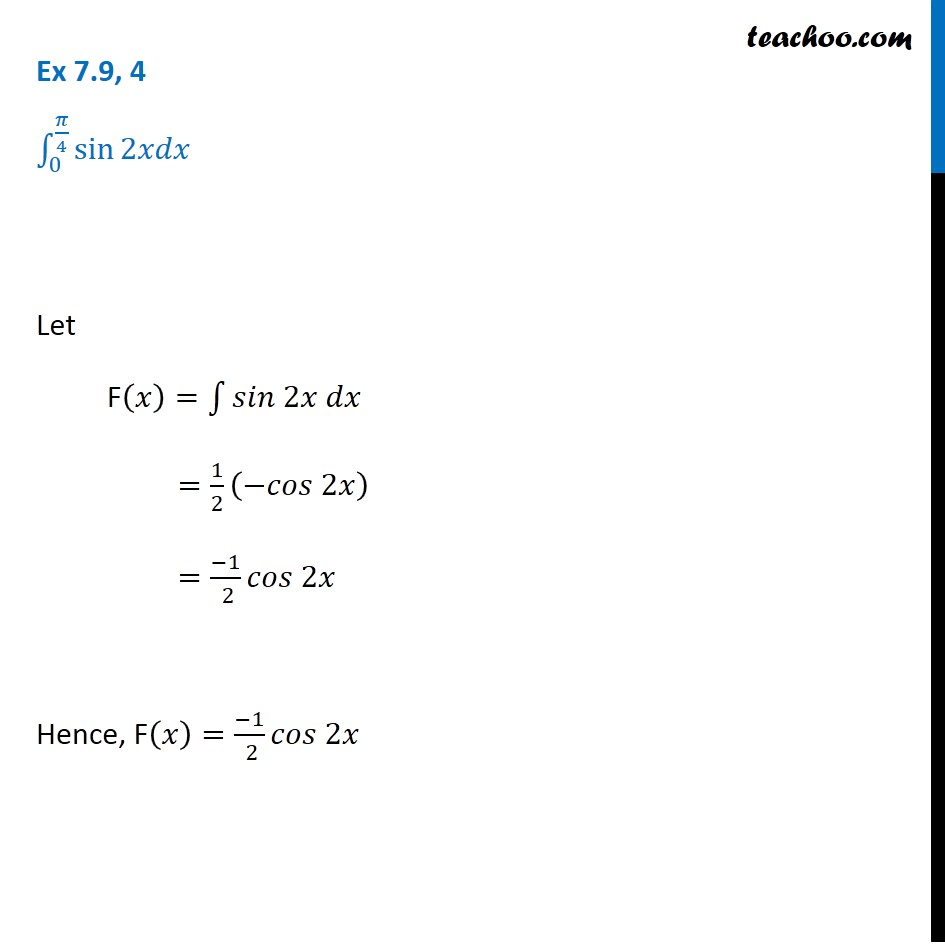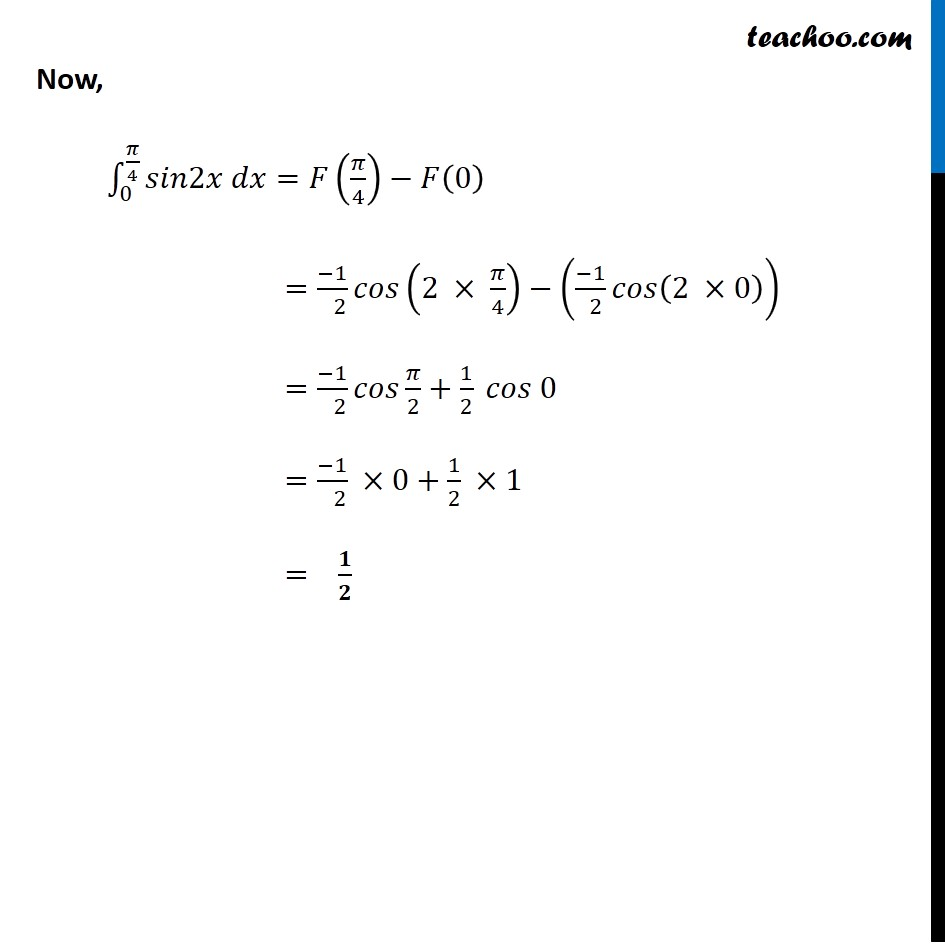Integration Full Chapter Explained - Integration Class 12 - Everything you need1. Chapter 7 Class 12 Integrals
2. Concept wise
3. Definite Integration - By Formulae

Transcript

Ex 7.9, 4 ∫_0^(𝜋/4)▒sin⁡2𝑥𝑑𝑥 Let F(𝑥)=∫1▒〖𝑠𝑖𝑛 2𝑥 𝑑𝑥〗 =1/2 (−𝑐𝑜𝑠 2𝑥) =(−1)/( 2) 𝑐𝑜𝑠 2𝑥 Hence, F(𝑥)=(−1)/( 2) 𝑐𝑜𝑠 2𝑥 Now, ∫_0^(𝜋/4)▒〖𝑠𝑖𝑛2𝑥 𝑑𝑥〗=𝐹(𝜋/4)−𝐹(0) =(−1)/( 2) 𝑐𝑜𝑠(2 × 𝜋/4)−((−1)/( 2) 𝑐𝑜𝑠(2 ×0)) =(−1)/( 2) 𝑐𝑜𝑠 𝜋/2+1/2 𝑐𝑜𝑠 0 =(−1)/( 2) ×0+1/2 ×1 = 𝟏/𝟐

Definite Integration - By Formulae

About the AuthorDavneet Singh
Davneet Singh is a graduate from Indian Institute of Technology, Kanpur. He has been teaching from the past 9 years. He provides courses for Maths and Science at Teachoo.